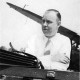## Quantifying the risk of random contact with infectious individuals

Posted: May 15, 2020 in probability
Tags: , , , , , ,

For citizens like me, old enough and perhaps wise enough to take a cautious view of mingling again with one’s fellow man after a period of lockdown in a time of pandemic, it’s natural to wonder about the risk of contact with infectious individuals as one re-establishes one’s daily routines. I thought this over and figured that if I knew the proportion of a population carrying infection, I could use probability theory to quantify the risk.

– – – –

How many random contacts are required to have a 50% chance of at least one contact with an infectious individual?

Knowing the answer to this question provides a way of relating a level of human interaction to a level of risk. And this little formula supplies the answer:where n is the number of random contacts and q is calculated as follows:N – is the number of people in the local population
a – is the number of people carrying infection

Example 1

You live in a city of 10 million people. Diagnostic testing projects that around 125,000 are carrying infection.Assuming uniform distribution of infection in the population, probability theory indicates that 55 random contacts with people in your city are required to have a 50% chance of at least one contact with an infectious individual.

Example 2

You are a storeworker in a town where it is estimated that 0.25% of the population is carrying infection. Assuming the same percentage applies to customers visiting your store:Probability theory indicates that 277 random contacts with people in your store are required to have a 50% chance of at least one contact with an infectious individual.

– – – –

Background to the formula

Calculating the probability of one of two possible outcomes in n independent trials is an application of probability theory known as a binomial experiment where p is the probability of one outcome (success) and q is the probability of the other (failure).

A simple formula exists for calculating the probability of at least one successful outcome in n trialsIn the present context, n is the number of random contacts and q is the probability of an individual chosen at random not carrying infection. Setting P(X≥1) = ½ and solving for nBy the change of base rule logab = logxb/logxawhere the log can be expressed to any base.

– – – –

The value of testing

Diagnostic testing is an important tool for controlling the spread of infection as the graph below helps to illustrate. If the level of infection in the population rises above 1 percent, the number of random contacts necessary to promote spread is not large. But below 1 percent the picture quickly changes as n climbs, with the likelihood of transmission diminishing accordingly.– – – –

P Mander, May 2020

1.FlowCoef says:
2.Quantifying the risk of random contact with infectious individuals — carnotcycle | Chet Aero Marine says: